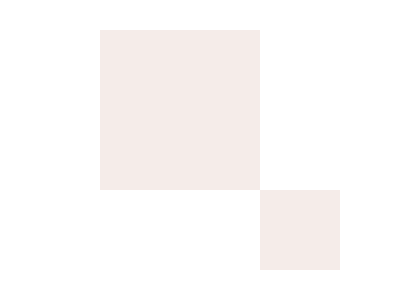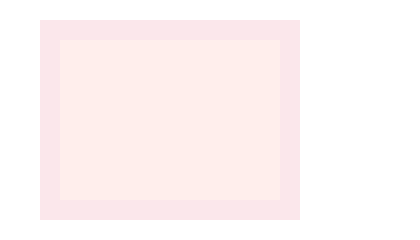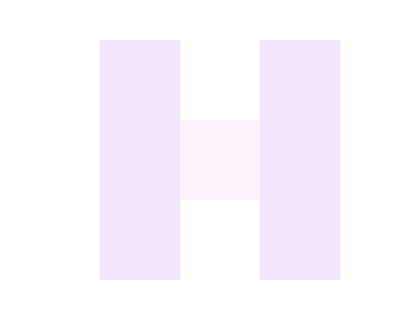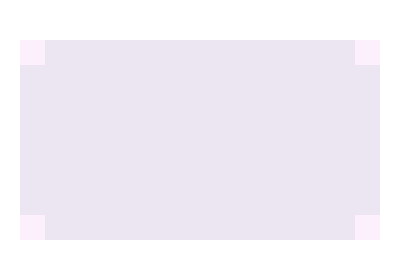# Variables

## Overview

Variables are containers used to store values.

To store a value in a variable, first declare the variable:

``var myVariable;``

Then, assign a value to it:

``myVariable = 42;``

We can also declare a variable and assign a value to it in one line:

``var myVariable = 42;``

If we declare a variable but don't assign a value to it, the value of the variable is `undefined`, which is an actual value we can check.

## Store and Use Values in Variables

In this example, we use variables to draw a small rectangle next to a larger rectangle. The larger rectangle is twice as wide and twice as tall as the small rectangle. We fill the small rectangle with the color `'BurlyWood'` and the larger rectangle with the color `Coral`.

We start by declaring and assigning values to the variables `x`, `y`, and `w`.

``````var x = 40;
var y = 20;
var w = 50;``````

We also declare and assign a value to the variable `h`, but instead of assigning it a number, we use an expression to calculate its value. Since the value of `w` is 50, the value of `1.5 * w` is 75, so the value of `h` is 75.

``var h = 1.5 * w;``

Changing the value of `w` after assigning the value of `1.5 * w` to `h` does not change the value of `h`. The value of `h` would still be 75 because it stores the value of the expression, not the expression itself.

We then use the values stored in `x`, `y`, `w`, and `h` to draw the small rectangle.

``context.fillRect(x, y, w, h);``

To draw the larger rectangle, we calculate and assign new values for our variables. For example, the x-coodinate of the larger rectangle is the x-coordinate of the small rectangle plus the width of the small rectangle, and the width of the larger rectangle is twice the width of the small rectangle.

``````x = x + w;
w = 2 * w;
h = 2 * h;``````

Note that we did not re-declare our variables before assigning new values to them. Variables should only be declared once.

Also, the order of these calculations and new assignments can be important. If we had assigned a new value to `w` before assigning a new value to `x`, the x-coordinate of the larger rectangle would be too far to the right.

When we draw the larger rectangle, we use the same variables, but all of them have new values except for the variable `y`.

``context.fillRect(x, y, w, h);``

By using variables to calculate its size and position, the larger rectangle is always next to the small rectangle and it is always twice as wide and twice as tall as the small rectangle. Change the intial values for `x`, `y`, and `w` to see what happens.

Quick Reference:

Message Log

## Challenge 1

Declare the variable `x` and assign it the value 60.

Declare the variable `y` and assign it the value 40.

Declare the variable `h` and assign it the value 150.

Declare the variable `w` and assign it the value of `2 * h`.

Then, use the `context.fillRect()` method to draw a rectangle at (`x`, `y`) with width `w` and height `h` filled with the color `'#483D8B'`.

Message Log
##### Canvas (your drawing will display here)## Challenge 2

Declare the variable `x` and assign it the value 100.

Declare the variable `y` and assign it the value 30.

Declare the variable `s` and assign it the value 160.

Use the `context.fillRect()` method to draw a square at (`x`, `y`) with side length `s` filled with the color `'#A0522D'`.

Then, use expressions to calculate and assign new values for `x`, `y`, and `s` to draw a square with half the side length as the first square positioned at the first square's bottom right corner.

Draw the second square using the `context.fillRect()` method, filling it with the same color `'#A0522D'`.

Message Log
##### Canvas (your drawing will display here)## Use Variable Expressions Directly as Values

In this example, we draw a forest green rectangle and then a lime green rectangle covering the bottom right quarter of the forest green rectangle.

We start by declaring and assigning values to the variables `x`, `y`, `w`, and `h` for the forest green rectangle:

``````var x = 50;
var y = 50;
var w = 200;
var h = 150;``````

After setting the fillStyle to `'ForestGreen'`, we use the `context.fillRect()` method to draw the forest green rectangle:

``context.fillRect(x, y, w, h);``

Then, instead of assigning new values to our variables for the lime green rectangle, we set the fillStyle to `'LimeGreen'` and simply draw the lime green rectangle using the `context.fillRect()` method by passing it variable expressions instead variables:

``context.fillRect(x + w / 2, y + h / 2, w / 2, h / 2);``

The x-coordinate of the lime green rectangle is the value of the variable expression `x + w / 2`, which is the x-coordinate of the forest green rectangle plus half its width. In JavaScript, mathematical expressions follow order of operations, so the division is evaluated before the addition.

The y-coordinate of the lime green rectangle is the value of the variable expression `y + h / 2`, and its width is the value of `w / 2` and its height is the value of `h / 2`.

Quick Reference:

Message Log

## Challenge 3

Declare and assign variables to draw a rectangle filled with the color `'Crimson'` at the coordinates (40, 20) with width 260 and height 200.

Use the `context.fillRect()` method to draw the rectangle.

Then, after setting the fillStyle to the color `'Tomato'`, draw a second rectangle over the first rectangle so the first rectangle forms a 20-pixel-wide border around the second rectangle. When using the `context.fillRect()` method to draw the second rectangle, use variable expressions for its x-coordinate, y-coordinate, width, and height.

Message Log
##### Canvas (your drawing will display here)## Challenge 4

Use variable expressions to draw the letter H by drawing two dark violet rectangles and one violet square.

The two dark violet rectangles have the same width and height. Their heights are three times as long as their widths. The dark violet rectangle on the left is positioned at (100, 40) and its width is 80.

The violet square has the same width as the two dark violet rectangles and is centered between them.

Message Log
##### Canvas (your drawing will display here)## Challenge 5

Use variable expressions to draw a rectangle filled with the color `'Indigo'` at position (20, 40) with width 360 and height 200, and four squares filled with the color `'Orchid'` with side length 25 inside each corner of the rectangle.

Message Log
##### Canvas (your drawing will display here)## Variable Names and Scope

Variable names are case-sensitive and may contain letters, underscores (_), dollar signs (\$), and numerals (0-9). However, variable names cannot start with a numeral and must not be a JavaScript reserved word. Here is a list of

Also, variable names must be unique. There must not be two variables with the same name within the same scope.

Variables are either global or local depending on where they are declared. Variables declared within a function are local. Variables declared outside of a function are global. Local variables have a local scope and can only be accessed from within the function where they were declared. Global variables, on the other hand, have a global scope and can be accessed from anywhere.

In this example, we declare the variables `x` and `y` in the global scope, the variables `x` and `z` in the local scope of `myFunctionA()`, and the variables `x` and `y` in the local scope of `myFunctionB()`.

It is perfectly fine to declare three different variables with the same name in three different scopes. If we try to access variable `x` inside `myFunctionA()`, the computer assumes we're trying to access the variable `x` declared locally in that function.

If, on the other hand, we try to access variable `y` inside `myFunctionA()`, because there is no variable `y` declared locally, the computer assumes we're trying to access the global variable `y`.

Trying to access variable `z` either from the global scope or inside `myFunctionB()` results in an error because variable `z` has only been declared inside `myFunctionA()`, where the global scope and `myFunctionB()` can't see it.

In most of our programs, we store references to the canvas and the canvas's context in the global variables `canvas` and `context`, respectively, because we typically need to access the canvas and its context inside many different functions.

Never assign a value to a variable that hasn't been declared. Undeclared variables are automatically given a global scope, and global variables should only be created deliberately.

Quick Reference:

Message Log

## Variables and Data Types

Variables can store more than numbers. Variables can store numbers, strings (text), booleans (`true` or `false`), `null` and `undefined` values, and objects (including arrays).

Javascript is considered a loosely typed language because a value's type can change depending on how it is being used.

For example, in JavaScript, we can add numbers and we can add strings. If `a = 10` and `b = 16`, then the value of `a + b` is `26`. And if `a = 'The fox'` and `b = ' is hungry'`, then `a + b` is `'The fox is hungry'`.

But what happens if `a = 10` and `b = ' is hungry'`? Instead of saying it can't add a number and a string, JavaScript will convert the number into a string, so the value of `a + b` will be the string `'10 is hungry'`.

While the ability to add strings and numbers is useful, sometimes the results can be unexpected. Be careful when converting between data types and try to be as explicit as possible.

Message Log

## Ready for the next lesson?

Next up, the "Functions" lesson >